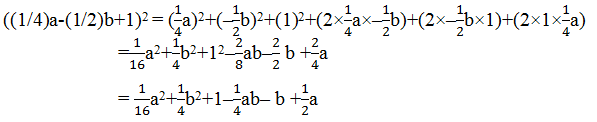Guru

# Expand each of the following, using suitable identities: (6) ((1/4)a-(1/2)b +1)2 Q.4(6)

• 0

What is the best way for solving the question of class 9th of Polynomials chapter of exercise 2.5 of math, give me the best way for solving this question in easy way how i solve it Expand each of the following, using suitable identities: (6) ((1/4)a-(1/2)b +1)2

Share

1. Using identity, (x+y+z)2 = x2+y2+z2+2xy+2yz+2zx

Here, x = (1/4)a

y = (-1/2)b

z = 1• 0